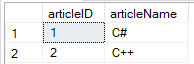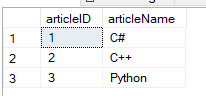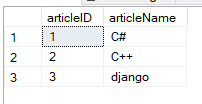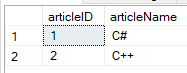# Basic Database Operations Using C#

In this article, you are going to learn about how to perform basic database operations using system.data.SqlClient namespace in C#. The basic operations are INSERT, UPDATE, SELECT and DELETE. Although the target database system is SQL Server Database, the same techniques can be applied to other database systems because the query syntax used is standard SQL that is generally supported by all relational database systems.

Prerequisites: Microsoft SQL Server Management Studio

Open Microsoft SQL Server Management Studio and write the below script to create a database and table in it.

```create database Demodb;

use Demodb;

CREATE TABLE demo(
articleID varchar(30) NOT NULL PRIMARY KEY,
articleName varchar(30) NOT NULL,
);

insert into demo values(1, 'C#');
insert into demo values(2, 'C++');
```

After executing the above script following table named demo is created and it contains the following data as shown in the screenshot.Connecting C# with Database: To work with a database, the first of all you required a connection. The connection to a database normally consists of the below-mentioned parameters.

• Database name or Data Source: The database name to which the connection needs to be set up and connection can be made or you can say only work with one database at a time.
• Credentials: The username and password which needs to be used to establish a connection to the database.
• Optional Parameters: For each database type, you can specify optional parameters to provide more information on how .NET should connect to the database to handle the data.

Note: Here, we are using command prompt to execute these codes. To see the result, you can use the Microsoft SQL Server Management Studio.

Code 1#: Connecting with database in C#

 `// C# code to connect the database ` `using` `System; ` `using` `System.Data.SqlClient; ` ` `  `namespace` `Database_Operation { ` ` `  `class` `DBConn { ` ` `  `    ``// Main Method ` `    ``static` `void` `Main() ` `    ``{ ` `        ``Connect(); ` `        ``Console.ReadKey(); ` `    ``} ` ` `  `    ``static` `void` `Connect() ` `    ``{ ` `        ``string` `constr; ` ` `  `        ``// for the connection to  ` `        ``// sql server database ` `        ``SqlConnection conn;  ` ` `  `        ``// Data Source is the name of the  ` `        ``// server on which the database is stored. ` `        ``// The Initial Catalog is used to specify ` `        ``// the name of the database ` `        ``// The UserID and Password are the credentials ` `        ``// required to connect to the database. ` `        ``constr = ``@"Data Source=DESKTOP-GP8F496;Initial Catalog=Demodb;User ID=sa;Password=24518300"``; ` ` `  `        ``conn = ``new` `SqlConnection(constr); ` ` `  `        ``// to open the connection ` `        ``conn.Open();  ` ` `  `        ``Console.WriteLine(``"Connection Open!"``); ` `   `  `        ``// to close the connection ` `        ``conn.Close();  ` `    ``} ` `} ` `} `

Output:

```Connection Open !
```

Code #2: Using Select Statement and SqlDataReader for accessing the data in C#

 `// C# code to demonstrate how  ` `// to use select statement ` `using` `System; ` `using` `System.Data.SqlClient; ` ` `  `namespace` `Database_Operation { ` ` `  `class` `SelectStatement{ ` ` `  `    ``// Main Method ` `    ``static` `void` `Main() ` `    ``{ ` `        ``Read(); ` `        ``Console.ReadKey(); ` `    ``} ` ` `  `    ``static` `void` `Read() ` `    ``{ ` `        ``string` `constr; ` ` `  `        ``// for the connection to  ` `        ``// sql server database ` `        ``SqlConnection conn;  ` ` `  `        ``// Data Source is the name of the  ` `        ``// server on which the database is stored. ` `        ``// The Initial Catalog is used to specify ` `        ``// the name of the database ` `        ``// The UserID and Password are the credentials ` `        ``// required to connect to the database. ` `        ``constr = ``@"Data Source=DESKTOP-GP8F496;Initial Catalog=Demodb;User ID=sa;Password=24518300"``; ` ` `  `        ``conn = ``new` `SqlConnection(constr); ` ` `  `        ``// to open the connection ` `        ``conn.Open();  ` ` `  `        ``// use to perform read and write ` `        ``// operations in the database ` `        ``SqlCommand cmd;  ` ` `  `        ``// use to read a row in ` `        ``// table one by one ` `        ``SqlDataReader dreader;  ` ` `  `        ``// to sore SQL command and ` `        ``// the output of SQL command ` `        ``string` `sql, output = ``""``;  ` ` `  `         ``// use to fetch rwos from demo table ` `        ``sql = ``"Select articleID, articleName from demo"``; ` ` `  `        ``// to execute the sql statement ` `        ``cmd = ``new` `SqlCommand(sql, conn);  ` ` `  `        ``// fetch all the rows  ` `        ``// from the demo table ` `        ``dreader = cmd.ExecuteReader();  ` ` `  `        ``// for one by one reading row ` `        ``while` `(dreader.Read()) { ` `            ``output = output + dreader.GetValue(0) + ``" - "` `+  ` `                                ``dreader.GetValue(1) + ``"\n"``; ` `        ``} ` ` `  `        ``// to display the output ` `        ``Console.Write(output);  ` ` `  `        ``// to close all the objects ` `        ``dreader.Close(); ` `        ``cmd.Dispose(); ` `        ``conn.Close(); ` `    ``} ` `} ` `} `

Output:

```1 - C#
2 - C++
```

Code #3: Inserting the data into the database using Insert Statement in C#

 `// C# code for how to use Insert Statement ` `using` `System; ` `using` `System.Data.SqlClient; ` `  `  `namespace` `Database_Operation { ` `     `  `class` `InsertStatement { ` `     `  `    ``// Main Method ` `    ``static` `void` `Main() ` `    ``{ ` `        ``Insert(); ` `        ``Console.ReadKey(); ` `    ``} ` `  `  `    ``static` `void` `Insert() ` `    ``{ ` `         ``string` `constr; ` `  `  `        ``// for the connection to  ` `        ``// sql server database ` `        ``SqlConnection conn;  ` `  `  `        ``// Data Source is the name of the  ` `        ``// server on which the database is stored. ` `        ``// The Initial Catalog is used to specify ` `        ``// the name of the database ` `        ``// The UserID and Password are the credentials ` `        ``// required to connect to the database. ` `        ``constr = ``@"Data Source=DESKTOP-GP8F496;Initial Catalog=Demodb;User ID=sa;Password=24518300"``; ` `  `  `        ``conn = ``new` `SqlConnection(constr); ` `  `  `        ``// to open the connection ` `        ``conn.Open();  ` `  `  `        ``// use to perform read and write ` `        ``// operations in the database ` `        ``SqlCommand cmd;  ` `         `  `        ``// data adapter object is use to  ` `        ``// insert, update or delete commands ` `        ``SqlDataAdapter adap = ``new` `SqlDataAdapter();  ` `         `  `        ``string` `sql = ``""``; ` `         `  `        ``// use the defined sql statement ` `        ``// against our database ` `        ``sql = ``"insert into demo values(3, 'Python')"``;  ` `         `  `        ``// use to execute the sql command so we  ` `        ``// are passing query and connection object ` `        ``cmd = ``new` `SqlCommand(sql, conn);  ` `         `  `        ``// associate the insert SQL  ` `        ``// command to adapter object ` `        ``adap.InsertCommand = ``new` `SqlCommand(sql, conn);  ` `         `  `        ``// use to execute the DML statement against ` `        ``// our database ` `        ``adap.InsertCommand.ExecuteNonQuery();  ` `         `  `        ``// closing all the objects ` `        ``cmd.Dispose(); ` `        ``conn.Close(); ` `    ``} ` `} ` `} `

Output:Code #4: Updating the data into the database using Update Statement in C#

 `// C# code for how to use Update Statement ` `using` `System; ` `using` `System.Data.SqlClient; ` `  `  `namespace` `Database_Operation { ` `     `  `class` `UpdateStatement { ` `     `  `    ``// Main Method ` `    ``static` `void` `Main() ` `    ``{ ` `        ``Update(); ` `        ``Console.ReadKey(); ` `    ``} ` `  `  `    ``static` `void` `Update() ` `    ``{ ` `       ``string` `constr; ` `   `  `        ``// for the connection to  ` `        ``// sql server database ` `        ``SqlConnection conn;  ` `   `  `        ``// Data Source is the name of the  ` `        ``// server on which the database is stored. ` `        ``// The Initial Catalog is used to specify ` `        ``// the name of the database ` `        ``// The UserID and Password are the credentials ` `        ``// required to connect to the database. ` `        ``constr = ``@"Data Source=DESKTOP-GP8F496;Initial Catalog=Demodb;User ID=sa;Password=24518300"``; ` `   `  `        ``conn = ``new` `SqlConnection(constr); ` `   `  `        ``// to open the connection ` `        ``conn.Open();  ` `   `  `        ``// use to perform read and write ` `        ``// operations in the database ` `        ``SqlCommand cmd;  ` `          `  `        ``// data adapter object is use to  ` `        ``// insert, update or delete commands ` `        ``SqlDataAdapter adap = ``new` `SqlDataAdapter();  ` `          `  `        ``string` `sql = ``""``; ` `         `  `        ``// use the define sql  ` `        ``// statement against our database ` `        ``sql = ``"update demo set articleName='django' where articleID=3"``;  ` `         `  `        ``// use to execute the sql command so we  ` `        ``// are passing query and connection object ` `        ``cmd = ``new` `SqlCommand(sql, conn);  ` `         `  `        ``// associate the insert SQL ` `        ``// command to adapter object ` `        ``adap.InsertCommand = ``new` `SqlCommand(sql, conn);  ` `         `  `        ``// use to execute the DML statement against  ` `        ``// our database  ` `        ``adap.InsertCommand.ExecuteNonQuery();  ` `         `  `        ``// closing all the objects ` `        ``cmd.Dispose(); ` `        ``conn.Close(); ` `    ``} ` `} ` `} `

Output:Code #5: Deleting the data into the database using Delete Statement in C#

 `// C# code for how to use Delete Statement ` `using` `System; ` `using` `System.Data.SqlClient; ` `  `  `namespace` `Database_Operation { ` `     `  `class` `DeleteStatement { ` `     `  `    ``// Main Method ` `    ``static` `void` `Main() ` `    ``{ ` `        ``Delete(); ` `        ``Console.ReadKey(); ` `    ``} ` `  `  `    ``static` `void` `Delete() ` `    ``{ ` `       ``string` `constr; ` `   `  `        ``// for the connection to  ` `        ``// sql server database ` `        ``SqlConnection conn;  ` `   `  `        ``// Data Source is the name of the  ` `        ``// server on which the database is stored. ` `        ``// The Initial Catalog is used to specify ` `        ``// the name of the database ` `        ``// The UserID and Password are the credentials ` `        ``// required to connect to the database. ` `        ``constr = ``@"Data Source=DESKTOP-GP8F496;Initial Catalog=Demodb;User ID=sa;Password=24518300"``; ` `   `  `        ``conn = ``new` `SqlConnection(constr); ` `   `  `        ``// to open the connection ` `        ``conn.Open();  ` `   `  `        ``// use to perform read and write ` `        ``// operations in the database ` `        ``SqlCommand cmd;  ` `          `  `        ``// data adapter object is use to  ` `        ``// insert, update or delete commands ` `        ``SqlDataAdapter adap = ``new` `SqlDataAdapter();  ` `          `  `        ``string` `sql = ``""``; ` `         `  `        ``// use the define SQL statement  ` `        ``// against our database ` `        ``sql = ``"delete from demo where articleID=3"``;  ` `         `  `        ``// use to execute the sql command so we  ` `        ``// are passing query and connection object ` `        ``cmd = ``new` `SqlCommand(sql, conn);  ` `         `  `        ``// associate the insert SQL  ` `        ``// command to adapter object ` `        ``adap.InsertCommand = ``new` `SqlCommand(sql, conn);  ` `         `  `        ``// use to execute the DML statement ` `        ``// against our database ` `        ``adap.InsertCommand.ExecuteNonQuery();  ` `         `  `        ``// closing all the objects ` `        ``cmd.Dispose(); ` `        ``conn.Close(); ` `    ``} ` `} ` `} `

Output:My Personal Notes arrow_drop_upCompetitive Programmer

If you like GeeksforGeeks and would like to contribute, you can also write an article using contribute.geeksforgeeks.org or mail your article to contribute@geeksforgeeks.org. See your article appearing on the GeeksforGeeks main page and help other Geeks.

Please Improve this article if you find anything incorrect by clicking on the "Improve Article" button below.

Article Tags :

Be the First to upvote.

Please write to us at contribute@geeksforgeeks.org to report any issue with the above content.